## HOW TO MAKE INFERENTIAL STATISTICS WITH SPSS

October 13 2018, By Chidi Rafael

When decisions or judgements are to be made from a study or a descriptive data, it is imperative to adopt various techniques for inferences.

One way to make inferences is by formulating a Hypothesis. A hypothesis may be needed in a study for users to decide appropriately on whether an assumption is true or false.

To make this tutorial simple and straight forward for a beginner, I will stick with these areas where Inferential Statistics could be applied in research. These four areas are:

1. Relationships.
2. Association.
3. Correlation.
4. Variance.

Before we go deep into these four major areas mentioned above, it is necessary to know the Decision Rule when Using the Statistical Package for Social Sciences (SPSS) for judgements.

Using a 95% Confidence Level, the decision is pegged at 0.05. (p =0.05). This simply implies that when p (the Significance level) is less than or equal to 0.05, we should accept (i.e. it is Significant Statistically).

On the other hand, when p is greater than 0.05, we should reject the hypothesis (i.e. it is not Significant Statistically).
The table below represents the Decision Rule:

 Result Decision p< or = 0.05 Accept (Significant) p> 0.05 Reject (Not Significant)

Figure 1.0
*This is dependent on a significance level of 0.05.

A) Relationships: Applying Linear Regression in SPSS
To measure relationship(s) between two or more variables linearly, we can use the Linear Regression. The example below illustrates how this can be applied using SPSS.

Illustration 1
The following data shows the Income and Expenditure pattern of Mr. Okoro from January to June 2018.

 MR OKORO INCOME AND EXPENDITURE REPORT FROM JAN-JUNE 2018 MONTH INCOME (NGN) EXPENDITURE (NGN) JAN 35,000 32,500 FEB 50,000 43,000 MAR 53,000 50,000 MAY 61,000 58,000 JUNE 74,000 73,000

Figure 2.0

Objective
To compute the data in Figure 2.0 in SPSS and test if there is a Significant Relationship between Income and Expenditure.

Solution to Illustration 1 Using SPSS

1. Open the SPSS Software and click on Variable View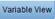2. Name your Variables (No spaces Accepted) as shown below: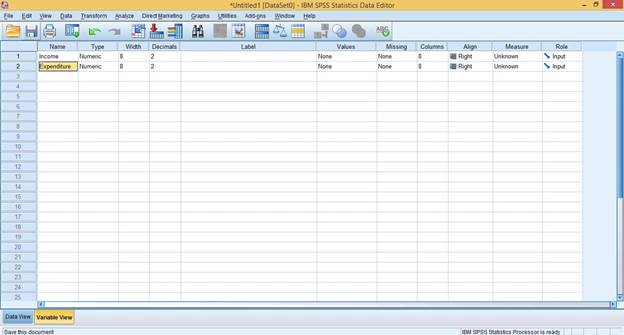Credit: IBM SPSS Statistics

1. Click on Data View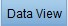button to enter the values or results gotten from the study. See below:Credit: IBM SPSS Statistics

1. Click on Analyze > Regression > Linear.
2. Move the Income Variable to the Independent(s) and Expenditure Variable to Dependent using the Arrow button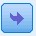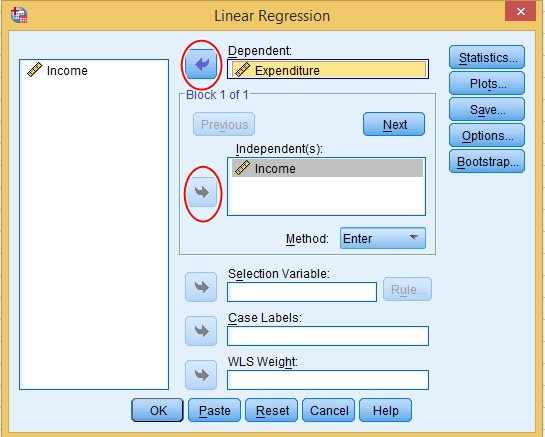Credit: IBM SPSS Statistics

1. Click OK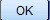for the result to display in the SPSS Output Screen.

Interpreting the Result
Our emphasis will be on 3 major tables:

1. Model Summary.
2. ANOVA.
3. Coefficients.Credit: IBM SPSS Statistics

Model Summary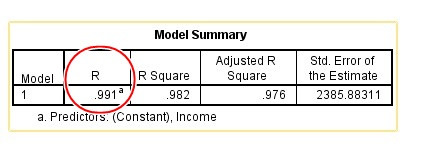The model Summary shows the relationship between the variables. R of 0.991 shows the strength of the relationship between the Independent Variable/Predictor (Income) and the Dependent Variable (Expenditure) in Decimal or Percentage form.

From the above example, Income accounts for 0.991 or 99.1% change in Expenditure. In other words, 99.1% change in Expenditure can be explained by the Predictor (Income).

ANOVA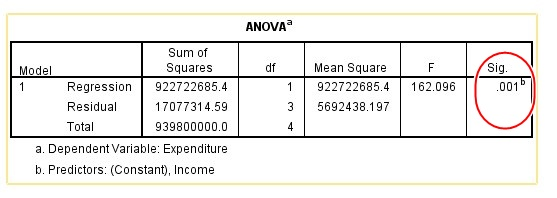The ANOVA table is used to check for Fitness of data in the model. To decide whether there is a statistically significant relationship between variables, we will look at the heighted in the Sig. Column above.

The Sig. Column of 0.001 is less than 0.05 (See Figure 1.0 for Decision Rule). This implies that there is a Statistically Significant relationship between Income and Expenditure.

Coefficients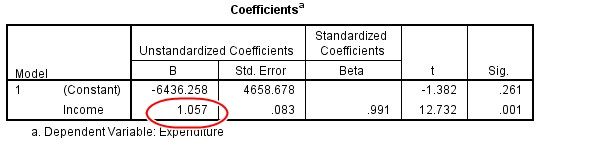The Coefficients table provides us with information to predict Expenditure from Income.
The highlighted above shows that 1 unit increase in Income will result to 1.057 increase in Expenditure.

B) ASSOCIATIONS: APPLYING CHI-SQUARE IN SPSS
Chi-Square can be used to test for Association between two or more variables. The Chi-Squared test measures if there is a significant difference between Expected Frequency (EF) and the Observed Frequency (OF).

Illustration 2
A survey was conducted on the reading preference of Undergraduate Students in The University of Abuja. With a Sample Size (n) of 20, the following results were gotten:

 Gender Preferred Reading Method Total Book Online Male 3 7 10 Female 6 4 10 Total 20

Figure 3.0

Objective
From the above data in Figure 3.0, You are required to compute the results in SPSS and test for Association between Gender and Preferred Reading Method using Chi-Square.

Solution to Illustration 2 in SPSS

1. Enter the Data (As shown in Figure 2.0) in the Data Viewas shown below: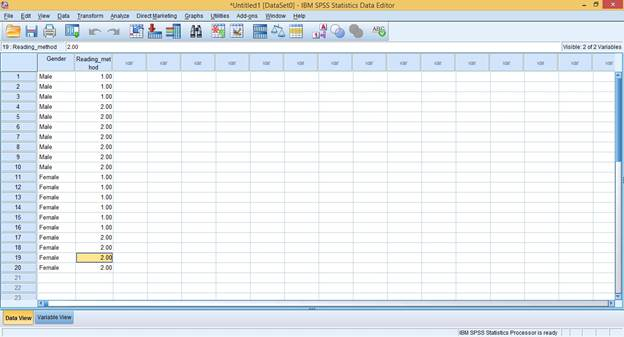Credit: IBM SPSS Statistics

NOTE: A little Data Coding is required in the Variable View. Data Type for Gender should be changed to ‘String’, and ‘1’ should be used to represent ‘Book’, 2 should represent ‘Online’.

1. Click on Analyze > Descriptive > Cross Tabs
2. Use the Arrow Buttonto move the Gender Variable to Row(s) and Reading_Method Variable to Column(S).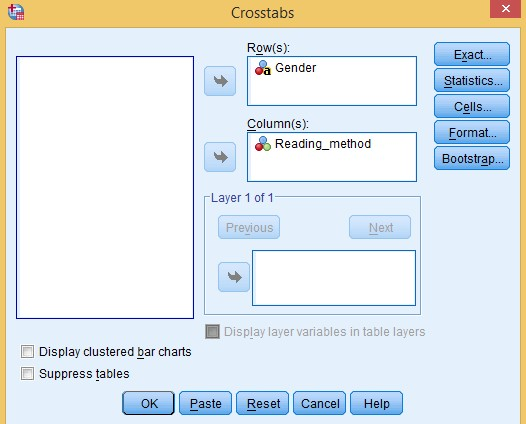Credit: IBM SPSS Statistics

1. Click on the Statistics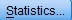button. Select Chi-Square and Phi and Cramer’s V, then Click on Continue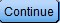.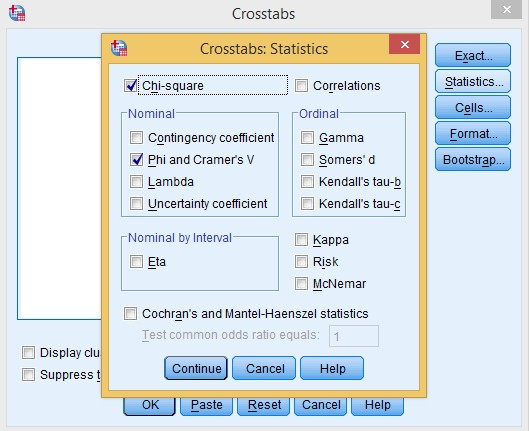Credit: IBM SPSS Statistics

1. Click on the Cells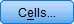button. Select Observed, Row Column and Total. Click Continue.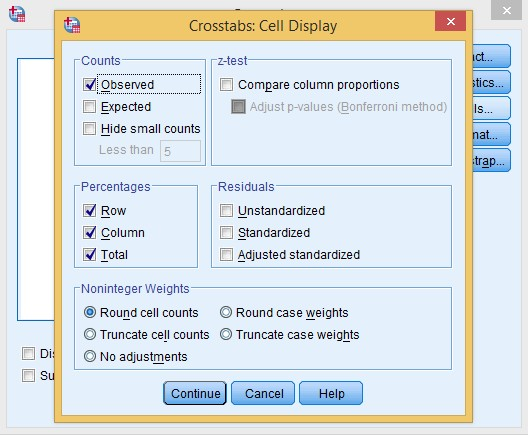Credit: IBM SPSS Statistics

1. Finally, Click the OKbutton for the Result.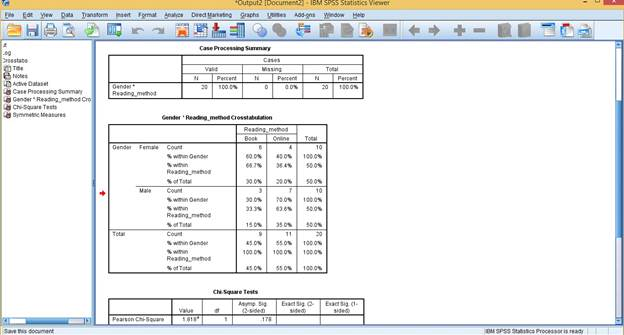Credit: IBM SPSS Statistics

Interpreting the Result
The Crosstabulation Table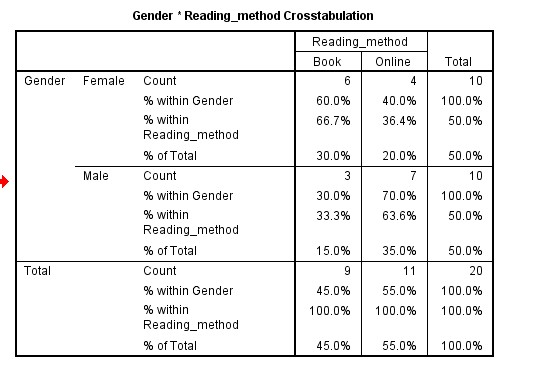This table simply illustrates the frequency distributions of Gender Versus Reading Method Preferences of the Respondents used for the study.

Chi-Square Tests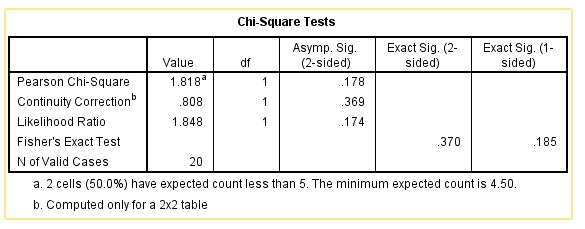This is the most important table used for measuring Association between variables used for the study.

From the table, the Pearson Chi-Square (p) = 0.178. since p > 0.05, we can conclude that there is no Statistically Significant association between Gender and the reading preference of Undergraduates in the University of Abuja.

C) APPLYING CORRELATION IN SPSS
The Pearson Correlation test measures the strength and direction of two variables. We will use the illustration below to demonstrate this.

Illustration 3
The following data shows the Income and Expenditure pattern of Mr. Okoro from January to June 2018.

 MR OKORO INCOME AND EXPENDITURE REPORT FROM JAN-JUNE 2018 MONTH INCOME (NGN) EXPENDITURE (NGN) JAN 35,000 32,500 FEB 50,000 43,000 MAR 53,000 50,000 MAY 61,000 58,000 JUNE 74,000 73,000

Figure 4.0

Objective
You are to compute the data in Figure 4.0 using SPSS and test if there is a Significant Correlation between Income and Expenditure.

Solution to Illustration 3 in SPSS

1. Properly Name your variables (Income and Expenditure) in the Variable View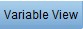and Enter your data on the Data Viewas shown below: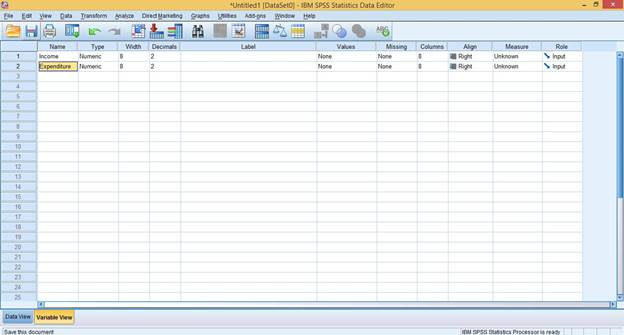Credit: IBM SPSS Statistics

1. Click on Analyze > Correlate > Bivariate
2. Use the Arrow buttonto move both variables to the Variables Box.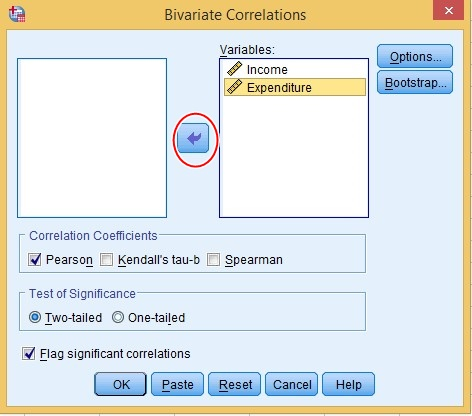Credit: IBM SPSS Statistics

1. Click OKInterpreting the Result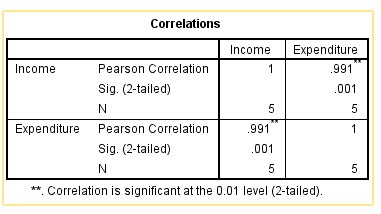The table above presents a mix of the Pearson Correlation, the Significance Value (Sig.) and the Sample Size (N) in a matrix form.

The Pearson Correlation (r) = 0.991 with p = 0.001. This implies that there is a significant Correlation between Income and Expenditure, since p < 0.05.

D) TEST FOR VARIANCE: APPLYING ONE WAY ANOVA IN SPSS
One way to test for variance is through One-Way ANOVA. One-way ANOVA compares the means between two or more variables to test for a statistically significant difference.

One-way ANOVA is most suitable for testing variance between two or more unrelated groups.

Illustration 4
A Quiz was set for two groups (Male and Female) in a class to determine if there is a significant difference between Gender and Academic Performance. With a sample size(n) of 10, the results below were gotten.

 Name Gender Score John Male 20.01 Mark Male 15.98 Andrew Male 43 Peter Male 21.4 Solomon Male 32.4 Joy Female 20.12 Amaka Female 13.5 Rose Female 56.1 Cynthia Female 18.7 Emem Female 45.2

Figure 5.0

Objective

You are Required to Compute the Data in Figure 5.0 using SPSS and test for Variance using the One-Way ANOVA.

Solution to Illustration 4 in SPSS

1. Enter the data properly in SPSS. You Data View should look like this: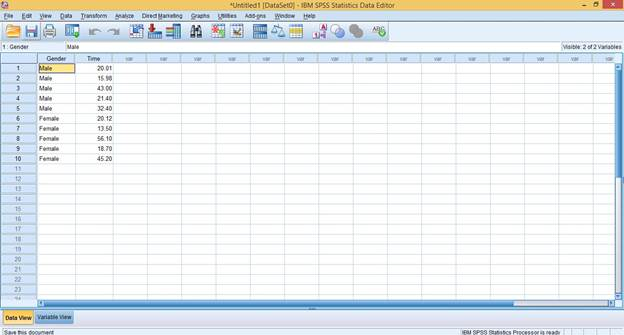Credit: IBM SPSS Statistics

1. Click on Analyze > Compare Means > One-Way ANOVA.
2. Use the Arrow Buttonto move the Dependent Variable (Score) to the Dependent List, and the Independent Variable (Gender) to the ‘Factor’ Field.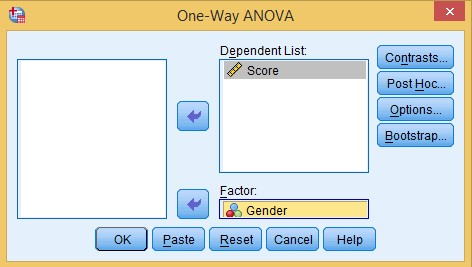Credit: IBM SPSS Statistics

1. Click on OK.

Interpreting the Result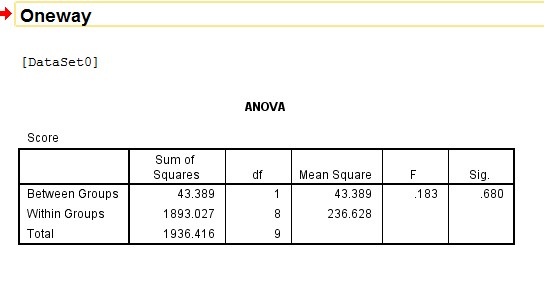The ANOVA table represents the results between Groups and within Groups used for the study.  p= 0.680 shows that there is no statistically significant difference between Gender and Academic Performance of students used for the study.